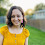## Thursday, February 9, 2012

### Parabolic Path of a Flying Q-Tip

This one I owe to one of my favorite teachers of all time.  It's a game we played in physics.  What you'll need for this is a drinking straw and 3-5 cotton swabs per student.

The lesson is on analyzing graphs of parabolas.  Since we just went over function transformations, we start with parabolas in the form f(x)=a(x-h)^2+k.  In this form, students seem to have very little trouble identifying the vertex.

So we graduate to f(x)=ax^2+bx+c.  Now, where's the vertex?  I used to do an elaborate presentation, completing the square for the general case and thus getting us back to the beautiful form above, and eloquently pointing out what h and k now are.  And then I came upon this beauty:

"Where is the x-coordinate of the vertex in relation to the two zeros?"  I ask.

From here, we have a little discussion, and eventually decide to average the two zeros to come up with the x-coordinate for the vertex, which turns out to be -b/2a (horrah!).

Now we practice.  I give the students a few quadratic functions and ask them to tell me all sorts of things about its graph.  The point I try to emphasize is that once you know the vertex and the direction the the parabola opens, you know a whole host of other information (such as the axis of symmetry, the min/max value and where it occurs, where the function is increasing/decreasing, and its range).

Now for the game.  I tell the students to take out the straws and Q-tips I passed out in the beginning of class.  I play this video and tell them whoever can shoot the cat with a Q-tip gets extra credit:

I gave out three Q-tips per students, but will most likely up that to four next semester, depending on how many students I have.  The students love this part of the lesson--the athletes in the room seem to take special pride in hitting the Nyan Cat.

And now back to the math.  We talk about how the path of their Q-tip takes the form a parabola.  We discuss if a should be positive or negative.  I then give them a model such as f(x)=-4x^2+4x+25 and explain that the path of their Q-tip could be modeled by such a function, where x is the time after the Q-tip's launch in seconds and f(x) is the Q-tip's height in inches.  (No, the model isn't perfect, but the numbers are fairly easy to work with and it gets the point across.)  We play with the model a bit, finding the ever-important vertex, among other things.

In the past, I've had students shoot at me during the lecture.  They love this.  Until they have to do the homework that night and realize they caught zilch during the lesson.  So, I tried the cat video this time.  It's probably not quite as entertaining, but in the long run, I think it may work better.

1.2.